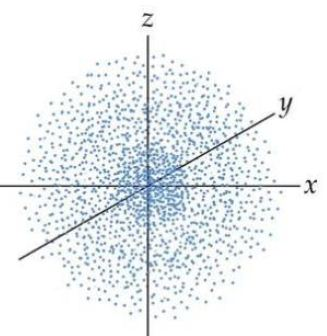Chemistry

# Quantum Mechanical Model

Bohr’s theory was a start but it only explained the hydrogen atom (one electron as a particle orbiting around a nucleus). The Quantum-Mechanical (QM) model, first developed by Erwin Schrodinger in 1926 describes electrons mathematically as both waves and particles. Schrodinger replaced Bohr’s orbits by complex 3-dimensional mathematical wave equations.

Orbitals: Orbitals are probability ‘maps because electrons do not have predictable positions. Heisenberg’s Uncertainty Principle -this states that the exact location and momentum of an electron is impossible to know at the same time. Whilst the value of one is known the value of the other remains unknown. For an electron in a particular energy level, the atomic orbitals can be imagined as specific locations (volumes of space) in which there is a high probability (>90% chance) of finding the electron. Each orbital can hold a maximum of 2 electrons. Orbitals describe the most likely place electrons will be found around the nucleus. We call this a probability density.Quantum Mechanical Model: Each electron in an atom can be uniquely described by four quantum numbers. A number, n, the Principal Quantum Number, is used to identify the main energy level in which the electrons are located, where n = 1, n = 2, n = 3 etc